AP Physics 2 Review
Below are a series of activities/problems that you can use to check your understanding of many of the topics and skills you will need to be familiar with for the AP Physics 2 Test. AP Review Videos here.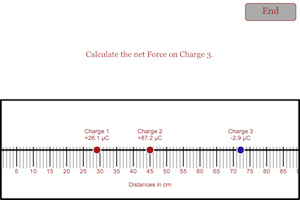Force Electric in a Line Problem Make sure you know how to find the net force on one of the charges in a line of charges. YouTube video describing the problem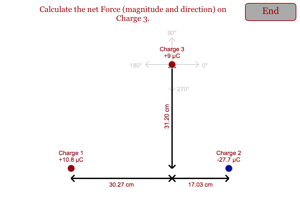Force Electric Triangular Arrangement Problem Make sure you can find the net force on one of the charges in a triangular arrangement of charges. YouTube video describing the problem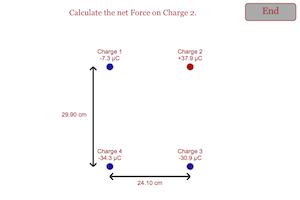Force Electric in a Box Problem Make sure you can find the net force on one of the charges in a rectangular arrangement of charges. YouTube video describing the problem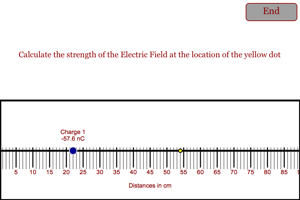Electric Field Simple Determine the electric field at a point in the vicinity of a charge. YouTube video describing the problem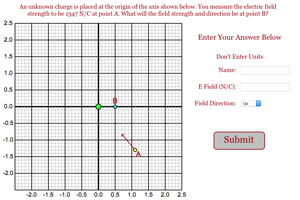Charge Causing Electric Field Determine the electric field at point B when when you know the electric field at point A. YouTube video describing the problem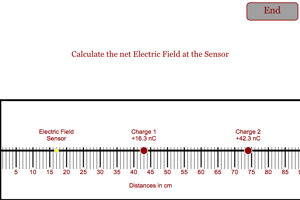Electric Field Line Determine the electric field at point on a line based on the values and location of two charges. YouTube video describing the problem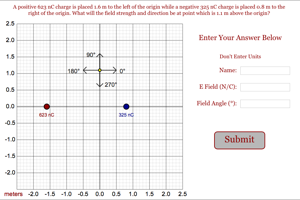Electric Field from Two Charges Determine the net electric field strength and direction that is caused by two different charges. YouTube video describing the problem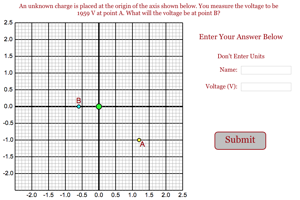Charge Causing Voltage Determine the voltage at point B when when you know the voltage at point A. YouTube video describing the problem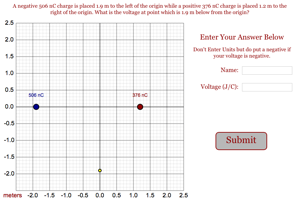Voltage From Two Charges Determine the net voltage from two charges. YouTube video describing the problem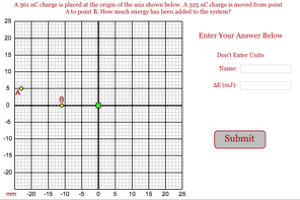Work Done in Moving Charge Determine the energy that must be added to a system to move a charge from one location to another in the vicinity of another charge. YouTube video describing the problem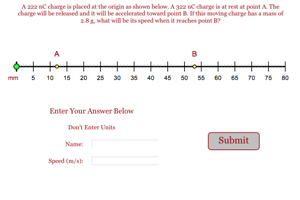Speed of Charge from Repulsion Determine the speed of a particle that has been repelled away from another particle with the same type of charge. YouTube video describing the problem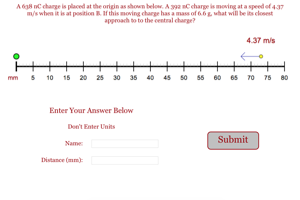Distance of Closest Approach In this problem you will be asked to find the closest approach between two charges. The charge at the origin is held at rest. The other charge is moving toward the charge with a certain speed and your job is to figure out how close the two charges will get before the moving charge stops and then begins moving in the opposite direction. YouTube video describing the problem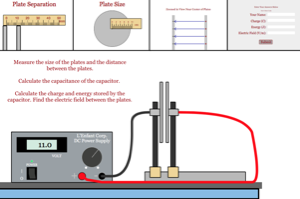Charge, Energy and Field on Capacitor In this problem you will be asked to determine the charge and energy stored on a capacitor. You will also find the electric field between the plates of the capacitor. YouTube video describing the problem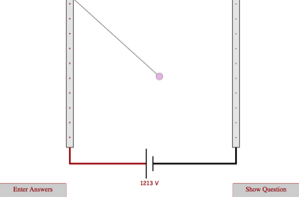Charge on String in Electric Field Students must determine the charge on a pith ball that is suspended in an electric field. YouTube video describing the problem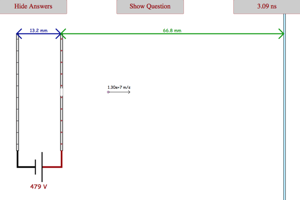Charge Fired by a Capacitor Students must determine the time it takes an electron to reach a phosphorescent screen when it is fired by a capacitor. Students must also find the top speed of the electron. YouTube video describing the problem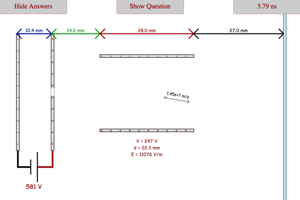Charge Deflected by a Capacitor Students must determine the time it takes an electron to reach a phosphorescent screen when it is fired by a capacitor. Students must also find the top speed of the electron and the amount of vertical deflection it experiences. YouTube video describing the problem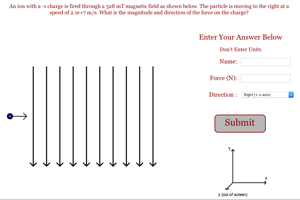Force on Charge Moving in a Magnetic Field In this problem you will be asked to find the force and the direction of the force on a charged particle moving through a magnetic field. YouTube video describing the problem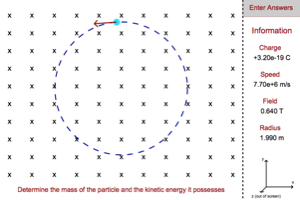Mass and KE of Particle in a Magnetic Field In this problem you will be asked to determine the mass and KE of a particle trapped in a magnetic field. You will be given the charge of the particle as well as the speed and strength of the field. YouTube video describing the problem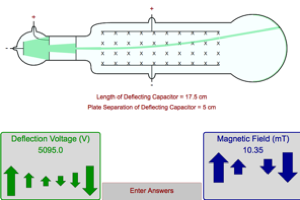Mass and Speed of Particle from Electric and Magnetic Fields In this problem you will be asked to determine the mass and speed of a particle being fired through variable magnetic and electric fields. This problem is done after students determine the charge of an electron using the Millikan Oil Drop Experiment. YouTube video describing the problem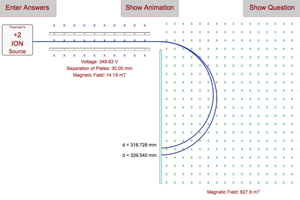Mass of Isotopes in Mass Spectrometer In this problem you will be asked to determine the mass of two different isotopes of an element based on their deflection by a magnetic field. YouTube video describing the problem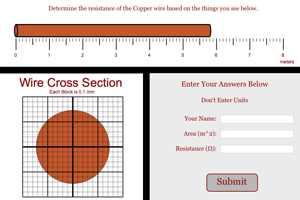Resistance of Wire Problem In this program you will need to determine the resistance of a wire based on its composition and its physical dimensions. You will be asked to give the area of the wire in square meters and the resistance of the wire in Ohms. YouTube video describing the problem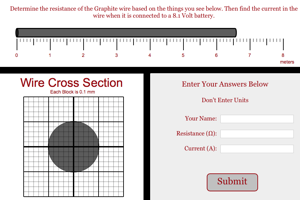Current in Wire Problem In this program you will need to determine the resistance of a wire based on its composition and its physical dimensions. You will be asked to find the current that would flow through the wire when the wire is connected to a certain voltage. YouTube video describing the problem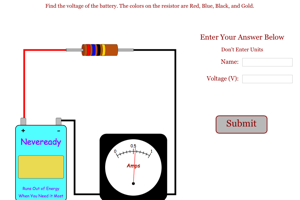Finding Battery Voltage Simple Determine the voltage of the battery based on the colors of the resistor and the current in the circuit. YouTube video describing the problem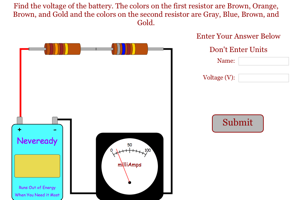Finding Battery Voltage Medium Determine the voltage of the battery based on the colors of the resistors and the current in the series circuit. YouTube video describing the problem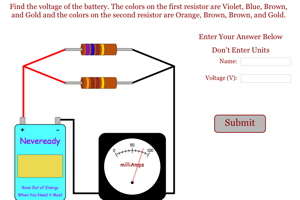Finding Battery Voltage Harder Determine the voltage of the battery based on the colors of the resistors and the current in the parallel circuit. YouTube video describing the problem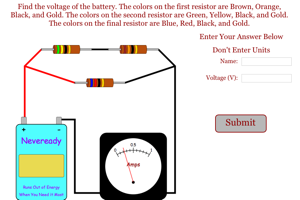Finding Battery Voltage Very Hard Determine the voltage of the battery based on the colors of the resistors and the current in a combination circuit. YouTube video describing the problem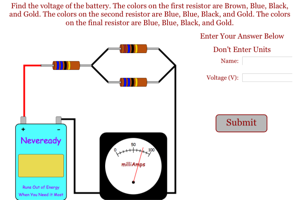Finding Battery Voltage Hardest Determine the voltage of the battery based on the colors of the resistors and the current in a combination circuit. YouTube video describing the problem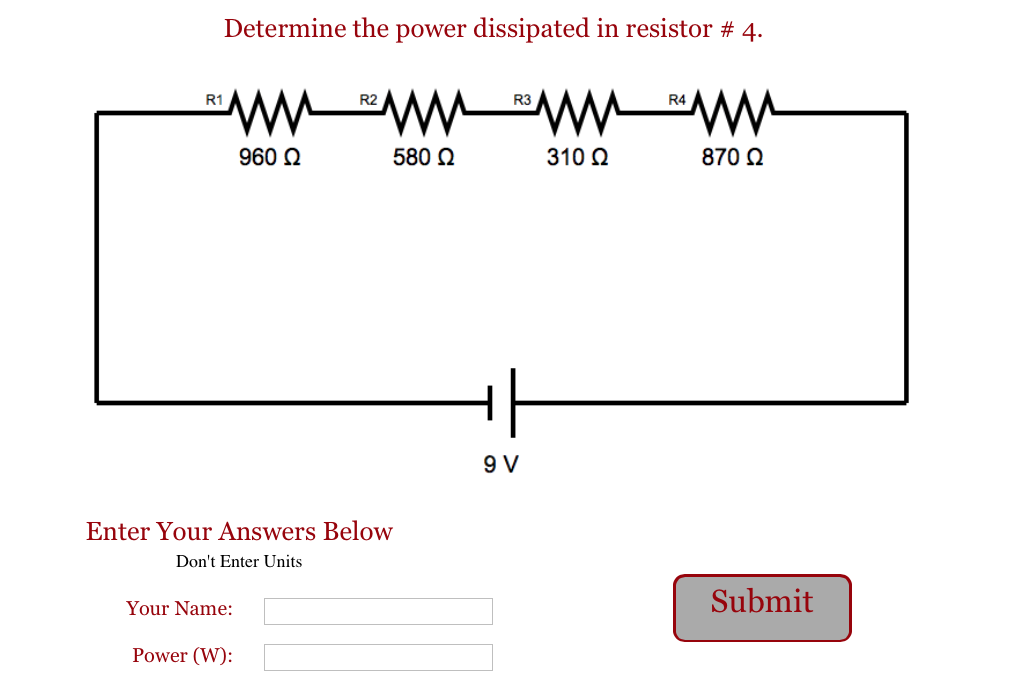Series Circuit Make sure you can determine the amount of power dissipated in a resistor that is part of a series circuit. YouTube video describing the problem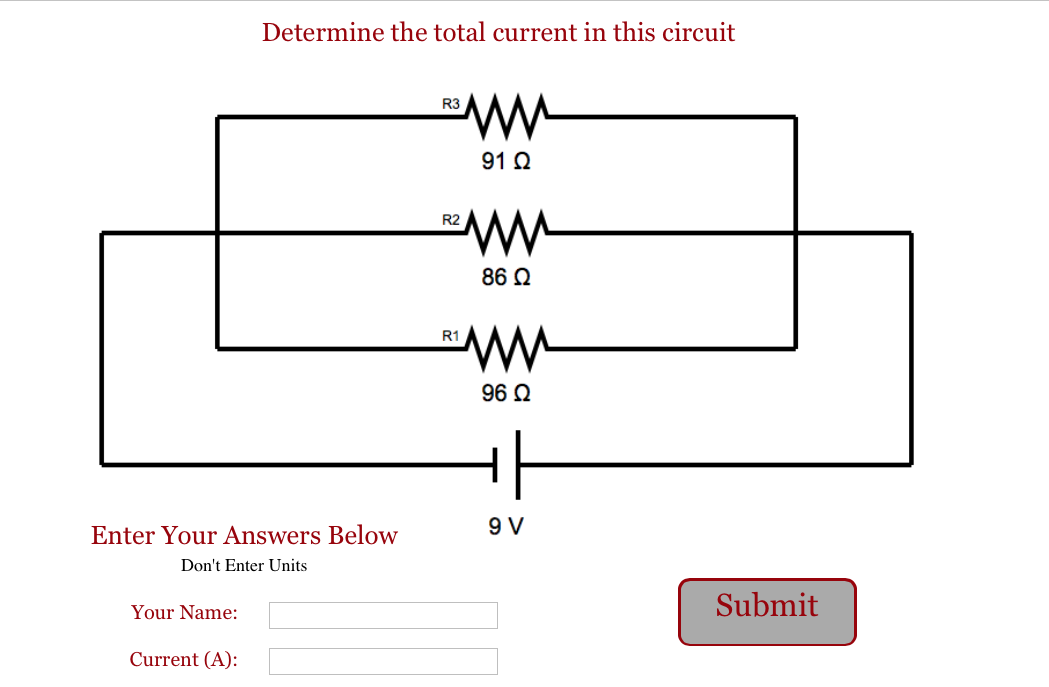Parallel Circuit Make sure you can determine the amount of current that is present in a parallel circuit. YouTube video describing the problem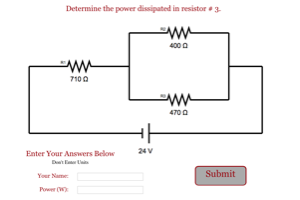Parallel in Series Circuit Make sure you can determine the amount of power dissipated in a resistor in a complex circuit. YouTube video describing the problem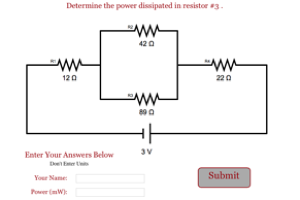Parallel in Double Series Circuit Determine the amount of power dissipated in a resistor in a complex circuit. YouTube video describing the problem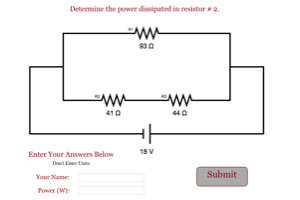Series in Parallel Circuit Make sure you can determine the amount of power dissipated in a resistor in a complex circuit. YouTube video describing the problem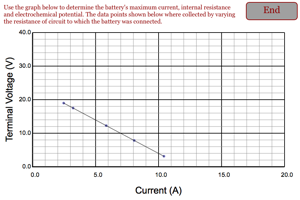Internal Resistance from Graph Determine the internal resistance of a battery based on the graph of terminal voltage vs. current. You must also find the maximum current and the electrochemical potential. YouTube video describing the problem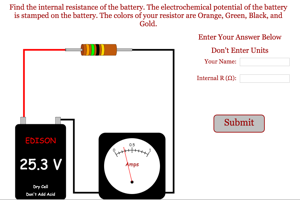Internal Resistance from Circuit Determine the internal resistance of a battery based on the electrochemical potential and the current that comes out of the battery when it is hooked to a resistor. YouTube video describing the problem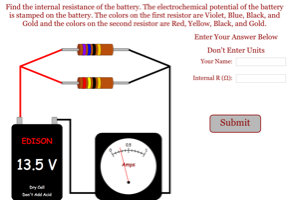Internal Resistance from Parallel Circuit Determine the internal resistance of a battery based on the electrochemical potential and the current that comes out of the battery when it is hooked to a series of resistors connected in parallel. YouTube video describing the problem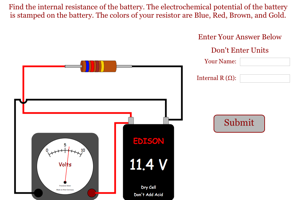Internal Resistance from From Terminal Voltage Determine the internal resistance of a battery based on the electrochemical potential and the voltage that is measured at the terminals of the battery when it is being used. YouTube video describing the problem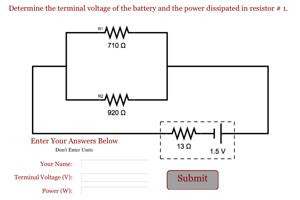Parallel Circuit with Internal Resistance This program will ask the students to find the terminal voltage of a battery with significant internal resistance. Students will also be asked to get the power of one of the external components for the circuit. YouTube video describing the lab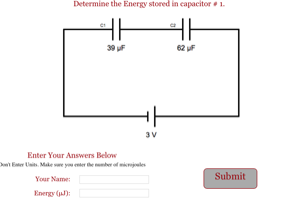Capacitors in Series Circuit Determine the amount of energy stored on a capacitor in a series circuit. YouTube video describing the problem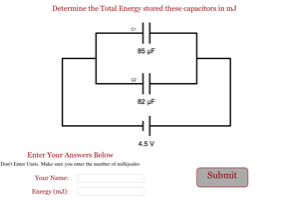Capacitors in Parallel Circuit Determine the total energy stored on a group of capacitor in a parallel circuit. YouTube video describing the problem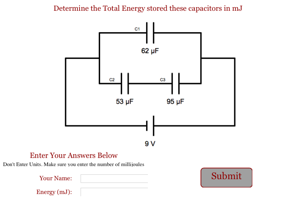Series of Capacitors in a Parallel Circuit Determine the total energy stored on a group of capacitor in a combination circuit. YouTube video describing the problem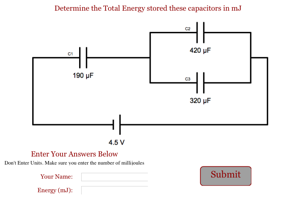Parallel of Capacitors in a Series Circuit Determine the total energy stored on a group of capacitor in a combination circuit. YouTube video describing the problem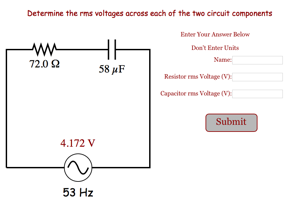RC Circuit Powered by DC Determine the initial current and final energy in an RC circuit powered by a DC source. YouTube video describing the problem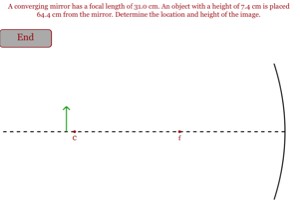Image From Converging Mirror Determine the height and location of the image formed by a converging mirror. YouTube video describing the problem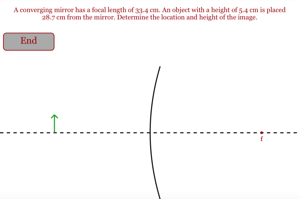Image From Diverging Mirror Determine the height and location of the image formed by a diverging mirror. YouTube video describing the problem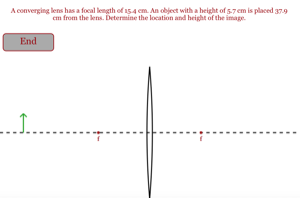Image From Converging Lens Determine the height and location of the image formed by a converging lens. YouTube video describing the problem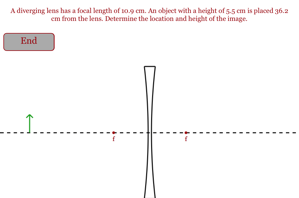Image From Diverging Lens Determine the height and location of the image formed by a diverging lens. YouTube video describing the problem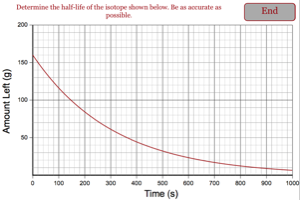Half-Life From Graph In this problem you must determine the half-life of an isotope based on the graph of the amount of the isotope left as a function of time. YouTube video describing the problem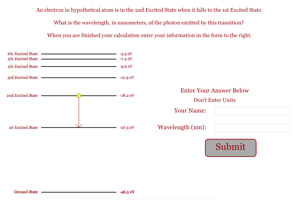Light From Atom In this problem you must determine the wavelength, in nanometers, of light emitted from an atom. YouTube video describing the problem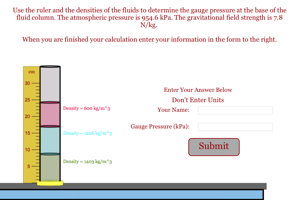Pressure from Fluid Column Students must determine the fluid pressure caused by a column of three immiscible fluids. YouTube video describing the problem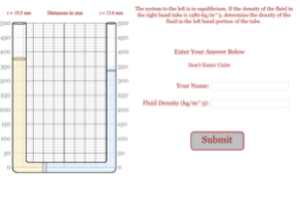Determining Density Students must determine the density of a fluid based on the fluid levels in a u-tube. YouTube video describing the problem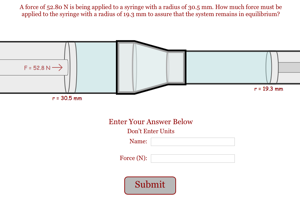Pascal's Principle Problem Students must determine the force required to hold a syringe in place when a second force is trying to get it to move. YouTube video describing the problem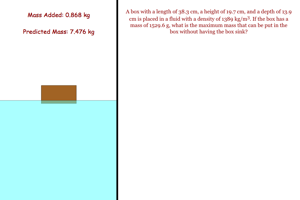Cargo Challenge Students must figure out how much mass can be put in a boat without having the boat go fully under the fluid. YouTube video describing the problem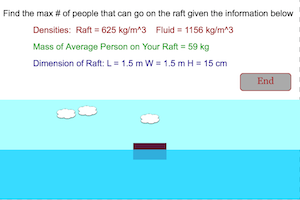Force Buoyancy Students must figure out the number of people that can go on a raft without having the raft go fully under the fluid. YouTube video describing the problem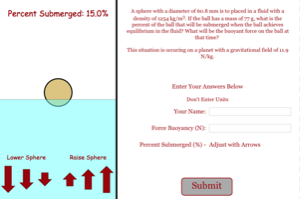Percent Submerged Challenge Students must figure out how much of a ball will be under a fluid when it is in equilibrium with its environment. YouTube video describing the problem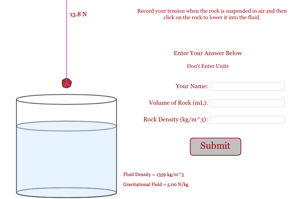Determining Density of Rock Students must determine the density of a rock based on the change in tension in the supporting string when the rock is lowered into a fluid. YouTube video describing the problem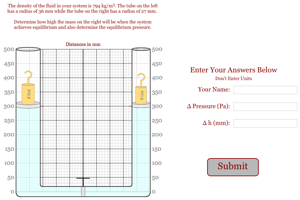Hydraulic Lift Problem Students must determine the difference in pressure that leads to the motion of masses in an hydraulic lift and the difference in height when the piston reaches equilibrium. YouTube video describing the problem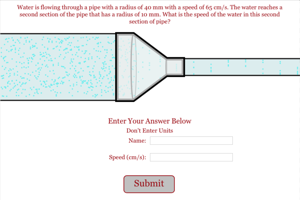Continuity of Flow In this problem you will have water flowing through two sections of piping with different diameter pipes joined by a coupler. You will be given the speed of the water in the first section of piping and you will need to find the speed in the second section. YouTube video describing the problem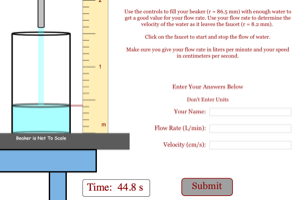Flow Rate Problem Students must determine the flow rate and speed of the water leaving a faucet based on the time it takes to fill a beaker to a certain height and the radius of the faucet the water is exiting from. YouTube video describing the problem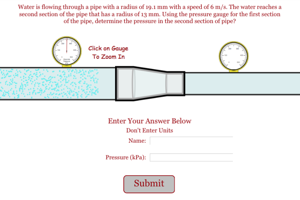Bernoulli's Principle In this problem you will have water flowing through two sections of piping with different diameter pipes joined by a coupler. The pressure gauge on one of the two pipes is broken. Your task is to find the pressure in the section of the pipe with the broken gauge. YouTube video describing the problem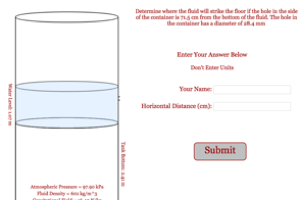Landing Location of Fluid In this problem you will have a tank of fluid get punctured and you will need to find the landing location of the fluid. YouTube video describing the problem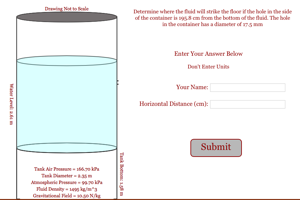Landing Location of Fluid with Different Pressures In this problem you will have a tank of fluid get punctured and you will need to find the landing location of the fluid. The tank in which the fluid is housed is pressurized above the value of the atmospheric pressure. YouTube video describing the problem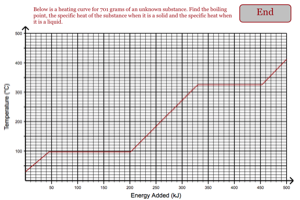Heating Curve Students must read a heating curve to determine what an object's boiling point is as well as its specific heat. Possible Student Directions YouTube of how to use the program.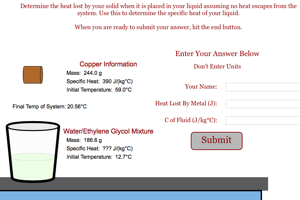Specific Heat of Fluid This program asks students to determine the specific heat of a fluid based on the amount of temperature change that occurs when a hot object is placed in the fluid. YouTube video describing the lab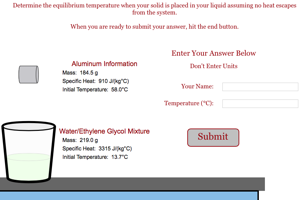Final Temperature of System Students must figure out the final temperature of a system when a hot object and a cold object are placed in a closed container. YouTube video describing the problem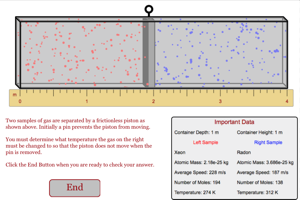Gas Partition Problem Determine the temperature required to keep a piston in equilibrium. YouTube video describing the problem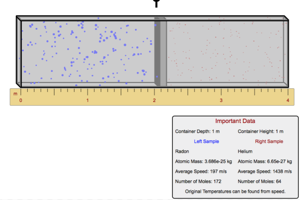Gas Partition Problem Level 2 Determine the temperature required to keep a piston in equilibrium. You must use information about the speed of the atoms in the left hand chamber to help you find the equilibrium pressure. YouTube video describing the problem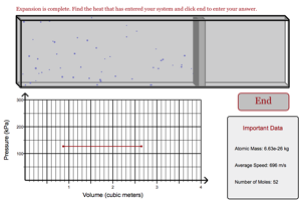Heat Added Isobaric Determine the heat that has been added to a gas in an isobaric process. YouTube video describing the problem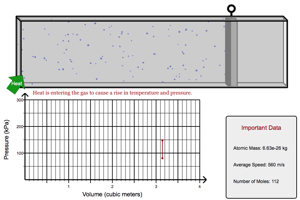Heat Added at Constant Volume Determine the heat that has been added to a gas in a process where the volume does not change. YouTube video describing the problem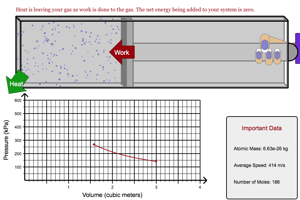Internal Energy in Isothermal Compression Determine the internal energy in a sample of gas that goes through an isothermal compression. YouTube video describing the problem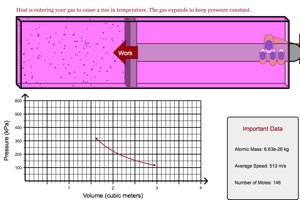Work Done in Adiabatic Compression Determine the work that was done when no heat enters or leaves the system. YouTube video describing the problem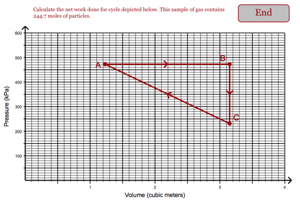Work Done in Cyclic Process (Triangle) Determine the net work that is done when a gas is brought through a simple three step process. Data is obtained from a PV Diagram. YouTube video describing the problem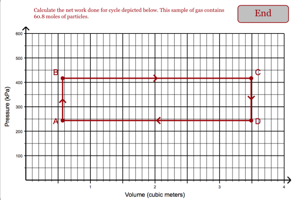Work Done in Cyclic Process Determine the net work that is done when a gas is brought through a simple process. Data is obtained from a PV Diagram. YouTube video describing the problem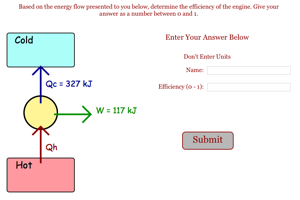Efficiency of Engine Determine the efficiency of an engine (0 - 1) based on the energy flow that is presented to you. YouTube video describing the problem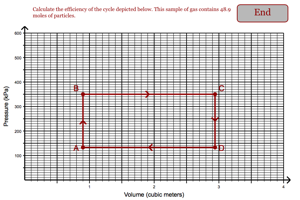Efficiency of Engine Based on PV Diagram Determine the efficiency of an engine (0 - 1) based on the PV Diagram that is presented to you. YouTube video describing the problem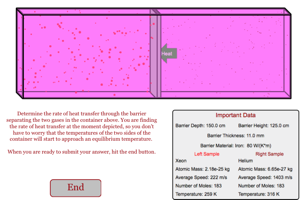Heat Transfer Rate Determine the rate at which heat moves through a barrier that is separating gases at two different temperatures. YouTube video describing the problem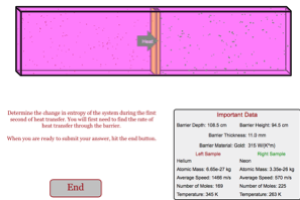Change in Entropy Determine the change in entropy that occurs when heat moves through a barrier that is separating gases at two different temperatures. YouTube video describing the problem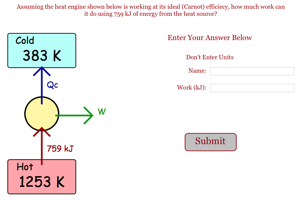Work Done in Carnot Engine Determine the work done by an engine working at it ideal (Carnot) efficiency. YouTube video describing the problem